# Physics 751 Homework #2

Due Friday September 12, 2008  11:00 am.

1. (a)  Defining the delta function as the limit of a narrow Gaussian wave packet (see the web notes on Fourier Series, etc.) prove it has the following properties: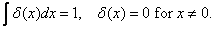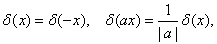(b)  Suppose you define the delta function by: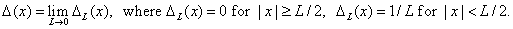Does this function have all the above properties?

2. Use Mathematica, Maple or Integral Tables to find the integral of (sinx)/x from 0 toand from 0 to infinity. Use your result to estimate the overshoot that appears in a Fourier series representation of a step function (Gibbs’ phenomenon).

3. Suppose at t = 0, a free particle of mass m, in one dimension, has a Gaussian wavefunctionBy taking a Fourier transform and putting in the explicit time-dependence for each plane wave component, find the form of the wavefunction as a function of time, and provide a physical interpretation in terms of finding the particle somewhere.

4. Denoting the lowest energy eigenstate in an infinite square well by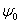, and the first excited state by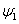, describe the behavior of the probability distribution as a function of time for the state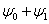(appropriately normalized).

Find the probability current at the midpoint of the well as a function of time.

How would your analysis be different for the state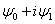?

5.  Prove Parseval’s Theorem:6.  Prove the rule for the Fourier Transform of a convolution of two functions: